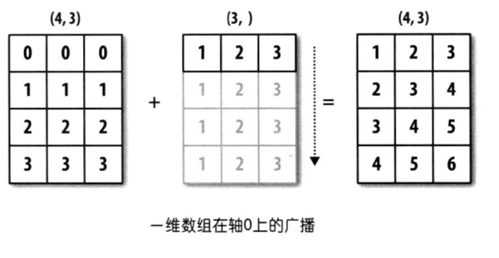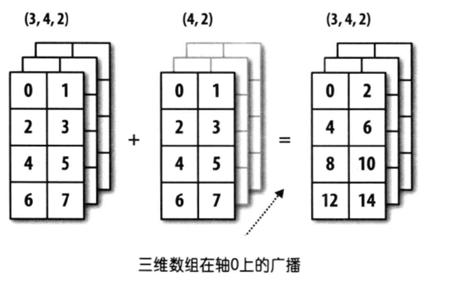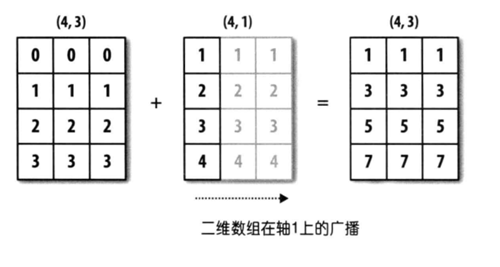# Numpy数组

## 1. 概述

• ndarray 数组要求数据类型一致，默认数据类型为 np.float64；显式更改数据类型需要使用 dtype 关键字。

## 2. axis 轴

Numpy 中 axis = n 对应 ndarray 的第 $n$[]，从最外层的 axis = 0，逐渐往内层递增。

## 3. 数组大小 & 维度

• ndarray 数组维度元组 shape 为从最外层到最里层逐层的大小；从最外层到最里层，对应 ndarray 数组的 axis 依次从 0 开始依次编号。

• ndarray.ndim ：数组维度数目

• ndarray.size ：数组所有元素数目 = 所有维度大小乘积

• ndarray.shape ：数组各个维度大小

## 4. 广播机制

Numpy 两个数组的相加、相减以及相乘都是对应元素之间的操作，当两个数组的形状并不相同时，Numpy 采用广播机制扩展数组使得二者形状相同。Numpy 广播机制原则：

• 数组维度不同，后缘维度（从末尾开始算起的维度）的轴长相符• 数组维度相同，其中一个轴长为 1## 5. 常用函数

• ndarray.max() ：取数组最大元素；若指定 axis = 选项，则将数组的那个维度 [] 压缩掉，即仅保留那个维度 [] 中的最大元素。

• ndarray.sum() ：计算数组中元素的累加和；若指定 axis = 选项，则将数组的那个维度 [] 压缩掉，即计算那个维度 [] 中的元素累加和。

• ndarray.reshape() ：更改数组每个维度大小，重新组织数据

## 6. 参考

• 《利用python进行数据分析》